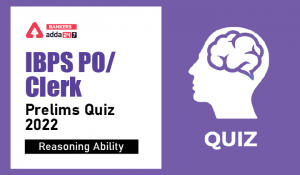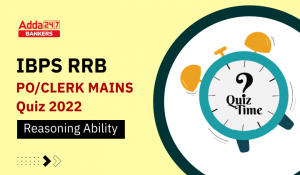Latest Banking jobs   »   SBI Clerk Prelims Reasoning Mini Mock-2:...

# SBI Clerk Prelims Reasoning Mini Mock-2: Puzzle, Inequality, Miscellaneous

SBI has released SBI Clerk Notification 2020 to recruit 8000+ candidates for the post of Junior Associate. As per the official notification, SBI Prelims Exam is to be held in the month of February and March. We will provide you daily reasoning quizzes and a study plan to enhance your preparation. Today’s Mini Mock-2 based on- Month based Puzzle, Inequality and Alphabet/Number based question.

Directions (1-5): Study the information carefully and answer the questions given below.
Seven persons J, K, L, M, N, O and P are going for holiday in seven different months of the same year i.e. January, March, April, May, June, July and August but not necessary in same order.
M goes in the month which has even number of days. One person goes between M and P. K goes after M. More than one person go between M and L. O goes just after L. Not more than two person goes between K and P. J does not go in the month which has odd number of day. N does not go in May.

Q1. In which of the following month P goes?
(a) June
(b) April
(c) August
(d) January
(e) None of these

Q2. Who of the following person goes on April?
(a) K
(b) M
(c) O
(d) P
(e) None of these

Q3. How many persons go between N and O?
(a) Three
(b) Two
(c) Four
(d) Five
(e) None of these

Q4. Four of the following five are alike in certain way based from a group, find the one that does not belong to that group?
(a) P
(b) K
(c) O
(d) M
(e) N

Q5. Which of the following person goes just before M?
(a) O
(b) P
(c) K
(d) J
(e) None of these

Q6. If all the letters in the word MEMORIZE are arranged in alphabetical order from left to right in such a way that vowels are arranged first followed by consonants, then how many letters are there in between I and R after the arrangement?
(a) Two
(b) None
(c) Three
(d) Five
(e) One

Q7. The position of how many alphabets will remain unchanged if each of the alphabets in the word ‘Firecracker’ is arranged in alphabetical order from left to right?
(a) Four
(b) Three
(c) None
(d) One
(e) Two

Q8. How many pairs of letters are there in the word “Prediction” each of which have as many letters between them in the word as they have between them in the English alphabetical series?
(a) One
(b) Two
(c) Three
(d) More than three
(e) None

Q9. In a certain code, ROOMMATE is written as NPPSFUBN. How is SCRABBLE written in the code?
(a) TDSBCCFM
(b) FMCCBSDT
(c) BSDTFMCC
(d) BSDZFMCC
(e) None of these

Q10. If in the number 463965812, 2 is added to each of the digit which is less than and equal to 5 and 3 is subtracted from each of the digit which is greater than five then all the numbers are arranged in decreasing order from right to left then how many digits position remain same in new arrangement?
(a) Three
(b) None
(c) One
(d) Two
(e) Four

Direction (11-15): In these questions, relationship between different elements is show in the statements. The statements are followed by conclusions. Study the conclusions based on the given statements and select the appropriate answer:
(a) If only conclusion I follows.
(b) If only conclusion II follows.
(c) If either conclusion I or II follows
(d) If neither conclusion I nor II follows.
(e) If both conclusions I and II follow.

Q11. Statements: Q>Y<O<C>L≥K=J>M
Conclusion:
I: Q<O
II: M<C

Q12. Statements: X<M≤D>T=R≤W<Z
Conclusion
I: D≥X
II: T<Z

Q13. Statements: W=P≥L=K≥J=V<N
Conclusion
I: K<W
II: W=K

Q14. Statements: A>S<B≥E>L≤Z>J=R
Conclusion
I: Z≥B
II: B>Z

Q15. Statements: V=G>H=N≥L<O=T
Conclusion
I: L<G
II: T>N

Solution

Solutions(1-5):
Sol.S1.Ans.(d)
S2.Ans.(b)
S3.Ans.(c)
S4.Ans.(d)
S5.Ans.(e)

S6. Ans.(c)
Sol. Original Word- MEMORIZE
After operation- EEIOMMRZ

S7.Ans(e)
Sol. Original word- FIRECRACKER
Obtained word-ACCEEFIKRRR

S8. Ans.(d)
Sol.S9.Ans(c)
Sol.S10.Ans(d)
Original no.- 463965812
Obtained no.-333455667

S11. Ans(b)
Sol. I: Q<O(False) II: M<C (True)

S12. Ans(b)
Sol. I: D≥X(False) II: T<Z(True)

S13. Ans(c)
Sol. I: K<W(False) II: W=K (False)

S14. Ans(c)
Sol. I: Z≥B(False) II: B>Z(False)

S15. Ans(a)
Sol. I: L<G(True) II: T>N (False)

Download the PDF of Reasoning Quiz for 16 February 2020

#### If you are preparing for SBI Clerk Prelims Exam, then you can also check out a video for Reasoning below:

You may also like to read:

Practice with Crash Course and Online Test Series for SBI Clerk prelims

SBI Clerk Mock Test Online Test Series

SBI Clerk Prelims 2020 Video Course

#### Congratulations!Download Hindu Review of October 2021: Free PDFIncorrect details? Fill the form again here

Download Hindu Review of October 2021: Free PDF

Thank You, Your details have been submitted we will get back to you.
•Reasoning Ability Quiz For IBPS Clerk/PO...
•Reasoning Ability Quiz For IBPS RRB PO C...
•Reasoning Ability Quiz For IBPS Clerk/PO...
•Reasoning Ability Quiz For IBPS RRB PO C...
•Reasoning Ability Quiz For IBPS Clerk Pr...
•Reasoning Ability Quiz For IBPS RRB PO P...